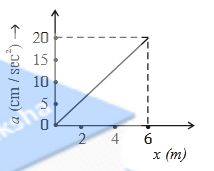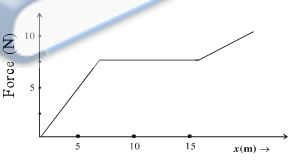11.

An 8 kg mass moves along the x-axis. Its accelerations as a function of its position are shown in the figure. What is the total work done on the mass by the force as the mass moves from x = 0 tox = 6cm?A. $$48\times10^-^3$$ J

B. $$98\times10^-^3$$ J

C. $$4.8\times10^-^3$$ J

D. $$9.8\times10^-^3$$ J

Explanation :

$$W= Fx = max =(m) (Area Covered by curve of a\rightarrow x graph)$$

12.

The work done by a force acting on a body is as shown in the graph. what is the total work done in covering an initial distance of15m?A. 50 J

B. 75 J

C. 100 J

D. 25 J

13.  A spring gun of spring constant $$90\times10^2$$ N/M is compressed 4cm by a ball of mass 16g. If the trigger is pulled, calculate the velocity of the ball.

A. 60 m/s

B. 3 m/s

C. 90 m/s

D. 30 m/s

Explanation :

Loss in P.E. of spring = gain in K.E. of ball

14.

A uniform chain of length 2m is kept on a table such that a length of 50cm hangs freely from the edge of the table. The total mass of the chain is 5kg. What is the work done in pulling the entire chain on the table? (g = 10 m\s2)

A. 7.2 J

B. 3 J

C. 4.6 J

D. 120 j

15.

A uniform chain of length L and mass M is lying on a smooth table and one-third of its is hanging vertically down over the edge of the table. If g is the acceleration due to gravity, the work required to pull the hanging part onto the table is

A. MgL

B. MgL / 3

C. MgL/9

D. MgL / 18

Explanation :

$$w=\frac{mgl}{2n^2}$$ (n=3 Given)

16.  A block of mass 5 kg is resting on a smooth surface. At what angle a force of 20N be acted on the body so that it will acquired a kinetic energy of 40J after moving 4m

A. $$30^0$$

B. $$45^0$$

C. $$60^0$$

D. $$120^0$$

Explanation :

$$Fd cos\Theta =\frac{1}{2}mv^2-\frac{1}{2mu^2}$$

17.  A cord is used to lower vertically a block of mass M by a distance d with constant down-word acceleration 9/2.work done by the cord on the block is

A. -Mgd/2

B. Mgd/4

C. -3Mgd/4

D. Mgd

18.  Natural length of a spring is 60 cm, and its spring constant is 2000N/m. A mass of 20kg is hung from it. The extension produced in the spring is...

A. 4.9 cm

B. 0.49 cm

C. 9.8 cm

D. 0.98 cm

$$\therefore x=\frac{F}{K}=\frac{mg}{k}$$